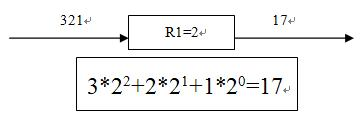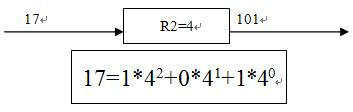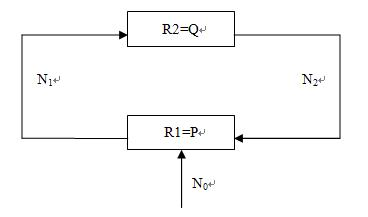Online JudgeProblem SetAuthorsOnline ContestsUser
Web Board
F.A.Qs
Statistical Charts
Problems
Submit Problem
Online Status
Prob.ID:
Register
Authors ranklist
Current Contest
Past Contests
Scheduled Contests
Award Contest
Register
Language:
Number System Converter
 Time Limit: 3000MS Memory Limit: 65536K Total Submissions: 1320 Accepted: 274

Description

ACM Lab produced a batch of number system converter recently. There are two kind of converter R1 and R2.

Given a parameter of P, converter R1's function is to transform the incoming P based integer into decimal number system. To simplify the design, R1 will not check that if the input is legal in P number system.R2 is similar to R1, while acting an inverse function: convert a decimal number to Q(specified by R2) number system.H.X.Q, a researcher of ACM Lab, made a circuit uses R1 and R2:(The circuit works at an order R1, R2, R1, R2 ...)

And she found an interesting phenomenon: if P<Q then for any input sequence N0, after plenty of convention N1 will finally equal to N2. For example, P=2, Q=4, N0=321, finally, N1=N2=3.

H.X.Q is excited for her discover, and wants to know what N1 and N2 will finally be in this circuit when P, Q and N0 is given.

Input

The input contains several test cases. The first line of each test case specifies two number P and Q (1 < P < Q < 37), follow by N0 (the length of N0 is not larger than 5,000,000). Note that a digit larger than 9 is given in small letters, with 'a'=10, 'b'=11 ...

Output

For each test case, output a line standing for the final sequence in the circuit.

Sample Input

```2 4
321
```

Sample Output

`3`

Source

[Submit]   [Go Back]   [Status]   [Discuss]Home PageGo BackTo top